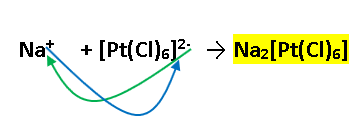# Problem: Give the formula of each coordination compound. Include square brackets around the coordination complex. Do not include the oxidation state on the metal. Use parentheses only around polyatomic ligands. For ethylenediamine, use (en) in the formula.a) sodium hexachloroplatinate(IV)b) dibromobis(ethylenediamine) cobalt(III) bromidec) pentaamminechlorochromium(III) chloride

###### FREE Expert Solution

We are asked to give the formula for the given coordination compounds.

Determine the identity of the complex and its counterion and follow the rules of the naming of coordination compounds.

a) sodium hexachloroplatinate(IV)

Step 1. Determine the anion and cation

• Anion complex appears to be hexachloroplatinate(IV) while the counterion is sodium

Step 2. Figure out the formula of the anion complex

hexachloro→ six Cl → (Cl)6

• For the charge of the complex, recall that:
• Charge of complex = charge of metal + total charge of ligands
• Metal Platinum has a charge of +4 from (IV)

Charge of complex = (+4) + (-1x6) = -2

• Anion complex will appear as: [Pt(Cl)6]-2

Step 3. Attach the counterion which is Na+

• Recall that for ionic compounds, the charge of the ion goes to the subscript of the counterion. For Na[Pt(CN)6]-292% (345 ratings)###### Problem Details

Give the formula of each coordination compound. Include square brackets around the coordination complex. Do not include the oxidation state on the metal. Use parentheses only around polyatomic ligands. For ethylenediamine, use (en) in the formula.

a) sodium hexachloroplatinate(IV)

b) dibromobis(ethylenediamine) cobalt(III) bromide

c) pentaamminechlorochromium(III) chloride